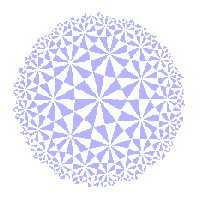# The Klein View of Geometry

subgroups and their geometries

 If we take E(2) and S(2) in matrix form, then we have the inclusions E(2) ≤ S(2) ≤ A(2). (see the affine group page). Then, the geometries are related in the manner indicated by Theorems K1 and K2. There, we noted that, in euclidean geometry, the congruence class containing the circle C0 = {(x,y) : x2 + y2 = 1} consists of all circles of radius 1. In similarity geometry, it consists of all circles, a union of euclidean classes. In affine geometry, it consists of all ellipses, a yet larger class. On the other hand, the set of lines is a single congruence class in each geometry, so not every class grows if we enlarge the group. Also, euclidean geometry has the peoperty of length of segments, similarity geometry has the property of ratio of lengths of segements, while affine has only the ratio of lengths of parallel segments. But all three geometries have the concept of betweenness. There are relations between other groups we have met, but these are not so simple as inclusion. Looking at them reveals some of the more subtle aspects of Klein's definitions. In the inversive group page, we met The ∞ Theorem S(2) is the subgroup of I(2) consisting of elements which fix ∞. There, S(2) was obtained by extending elements of S(2) to E+. Alternatively, we can define S(2) as the subgroup of I(2) fixing ∞ Then the argument for the ∞ Theorem shows that the restrictions of elements of S(2) to E give the group S(2). Note that S(2) is not itself a subgroup of I(2). Provided that we are careful about this, we can still derive information about similarity geometry from inversive geometry. As an example, we can give another proof of The Fundamental Theorem of Similarity Geometry If L = (A,B) and L' = (A',B') are lists of distinct points of E, then there is a unique element of S+(2) which maps L to L'. Alternative Proof From the Fundamental Theorem of Inversive Geometry, there is a unique element t of I+(2) mapping (A,B,∞) to (A',B',∞). Now t(∞) = ∞, so t ε S(2). Then s, the restriction of t to E, is in S(2). Since t is direct, so is s, i.e. s ε S+(2), as required. To see that s is unique, we observe that, if u maps L to L', then the extension maps L to L' and ∞ to ∞, so must be t. Likewise, the congruence classes are related. In inversive geometry, the i-lines form a single I(2)-congruence class. Elements of S(2) map ∞ to ∞, so map circles to circles and extended lines to extended lines. Restricting to E, we have two S(2)-congruence classes, circles and lines. other examples